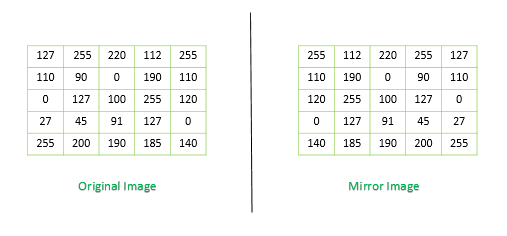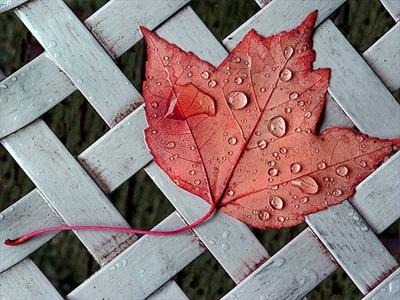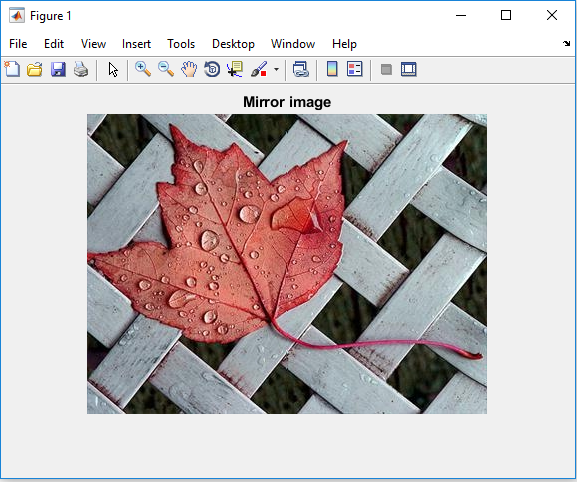# Create Mirror Image using MATLAB

Prerequisite: Image representation in MATLAB

In MATLAB, Images are stored in matrices, in which each element of the matrix corresponds to a single discrete pixel of the image. We can get the mirror image of the given image if we reverse the order of the pixels (elements of the matrix) in each row.Code #1: Using MATLAB Library function

 `% Read the target image file ` `img = imread(``'leaf.png'``); ` ` `  `% Reverse the element in each row ` `mirror_image = flip(img, 2); ` ` `  `% Display the mirror image ` `imshow(mirror_img);  ` `title(``'Mirror image'``); `

Code #2: Using matrix manipulation

 `% Read the target image file ` `img = imread(``'leaf.png'``); ` ` `  `% Flip the columns left to right ` `mirror_image = img(:, ``end` `:, -1:, 1, :); ` ` `  `% Display the mirror image ` `imshow(mirror_img);  ` `title(``'Mirror image'``);           `

Code #3: Using matrix manipulation (Using loops)

Approach:

• Read the source image file in MATLAB environment
• Get the Dimensions of the image matrix
• Reverse the order of the elements of each row in every image plane
• Display the mirror image

Below is the implementation of the above approach:

 `% Read the target image file ` `img = imread(``'leaf.png'``); ` ` `  `% Get the dimensions of the image ` `[x, y, z] =size(img); ` ` `  ` `  `% Reverse elements of each row ` `% in every image plane ` `for` `plane = 1: z ` `    ``for` `i = 1 : x ` `        ``len = y;  ` `        ``for` `j = 1 : y ` ` `  `            ``% To reverse the order of the element ` `            ``% of a row we can swap the  ` `            ``% leftmost element of the row with ` `            ``% its rightmost element   ` `     `  `            ``if` `j < y/2  ` `                ``temp = img(i, j, plane); ` `                ``img(i, j, plane) = img(i, len, plane); ` `                ``img(i, len, plane) = temp; ` `                ``len = len - 1; ` `            ``end` `        ``end` `    ``end` `end` ` `  ` `  `% Display the mirror image ` `imshow(img);  ` `title(``'Mirror image'``); `

Input Image: ` leaf.png`Output:My Personal Notes arrow_drop_upCheck out this Author's contributed articles.

If you like GeeksforGeeks and would like to contribute, you can also write an article using contribute.geeksforgeeks.org or mail your article to contribute@geeksforgeeks.org. See your article appearing on the GeeksforGeeks main page and help other Geeks.

Please Improve this article if you find anything incorrect by clicking on the "Improve Article" button below.

Article Tags :

Be the First to upvote.

Please write to us at contribute@geeksforgeeks.org to report any issue with the above content.